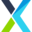Updated: March 5, 2021
###### What is a Pip?

Pips Are Small Measure of Change in Currency Pair Quotes or Prices

Pip is an abbreviation for point in percentage, and the smallest change in value a currency can make. A pip is in most cases equal to 1 basis point, which is  0.01 of one percent (one, one-hundredth of one percent).

An example of a currency pair where a pip is one basis point, is the GBP/USD. Take a look at the following image: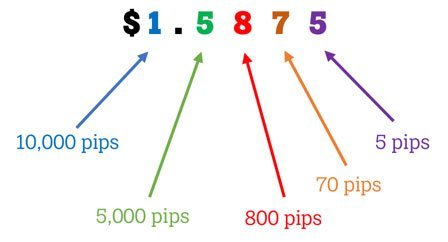In this example, the GBP/USD is trading at 1.5875, which means that one pound is worth \$1.5875. Here you can see that a pip is measured in the fourth decimal place, which is one basis point of a dollar.

The EUR/USD is quoted in the same way as the GBP/USD. Here is an example of how a price movement of 1 pip (up or down) would influence the price of this pair: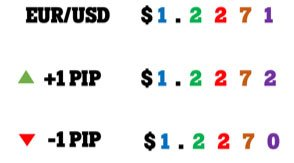Japanese yen pairs are not quoted with 4 or 5 decimals. An example is the USD/JPY. Here, pips are counted in the second decimal place. Look at this example:

###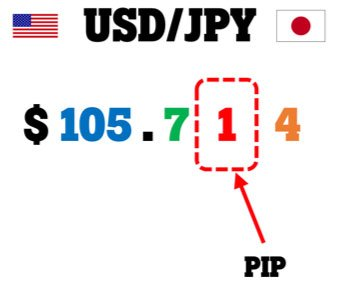If you need a pip calculator, you can find one here.

## Pips, Points, and Pipettes

Most forex brokers use a 5-decimal quotation system on pairs like the GBP/USD, EUR/USD, USD/CAD, etc. The fifth decimal place denotes 0.1 or 1/10 of a pip, which is also called a pipette or a point. Here is a visual example: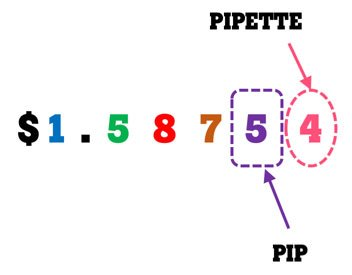## How to calculate the Pip value of a Currency pair?

To keep it simple for now, let’s assume the base currency of your trading account is U.S. dollar and you’re trading micro lots (0.01 lots). Let’s look at three different types of currency pairs:

1. Currency pairs wherein the U.S. dollar is the quote (2nd) currency.

For example, the EUR/USD, GBP/USD, AUD/USD, and the NZD/USD. With each of these pairs, the pip value is always \$0.10 for a micro lot (0.01 lots).

How do we know this? The pip value on these pairs is situated in the fourth decimal place at \$0.0001. The volume of a micro lot is 1,000 units of the currency pair. To calculate the lot size in U.S. dollar, multiply the volume (1000) by the pip value (\$0.0001) to get \$0.10.

1. Currency pairs wherein the U.S. dollar is the base (1st) currency

For example, the USD/JPY, USD/CHF, USD/ZAR, USD/CAD, USD/NOK, USD/SEK, etc. In this case, the pip value in USD is not constant, but fluctuates with the exchange rate.

With pairs like these, you can take the following steps:

• Calculate the pip value in the quote currency (the second currency).
• Convert this pip value to U.S. dollar with the exchange rate of that pair.

Here is an example of how to calculate the pip value of the USD/JPY when the exchange rate is 125.00 Japanese yen for one U.S. dollar:

Step 1: One micro lot is 1,000 units if the pair. This is the volume. With the USD/JPY, a pip is situated in the second decimal place at 0.01. To calculate the pip value in yen, multiply the volume (1000) by the pip value (0.01) to get 10 yen.

Step 2: Convert the pip value of 10 yen to the equivalent U.S. dollar value by dividing 10 yen by the current exchange rate of 125.

10/125 = 0.08 U.S. dollar per pip.

1. Pairs that don’t contain the U.S. dollar

For example, the EUR/JPY, GBP/JPY, EUR/NZD, CAD/JPY, CHF/JPY, EUR/SEK, EUR/GBP, etc.

With pairs like these, you can take the following steps:

• Calculate the pip value in the quote currency (the second currency)
• Convert this pip value to U.S. dollar with the specific currency pair that combines the USD and the quote currency of the pair you’re trying to get the pip value of.

Here is an example of how to calculate the pip value (in USD) of the GBP/NZD when the exchange rate is 1.95000 New Zealand dollar for one British pound and the NZD/USD exchange rate is 0.70000:

Step 1: One micro lot is 1,000 units if the pair. This is the volume. With the GBP/NZD, a pip is situated in the fourth decimal place at 0.0001. To calculate the pip value in NZD, multiply the volume (1000) by the pip value (0.0001) to get 0.10 NZD. Because the NZD is the quote currency, the pip value remains constant at 0.10 NZD.

Step 2: Convert the pip value of 0.10 NZD to the equivalent U.S. dollar value. To do this, keep in mind that we now need the NZD/USD exchange rate to determine how many U.S. dollars we can get for 0.10 New Zealand dollar.

The NZD/USD exchange rate is 0.70000 USD for one NZD, which means that our pip cost of 0.10 NZD is equal to 0.07 U.S. dollar. (We simply multiply 0.1 by 0.7).

Can you see that the GBP/NZD exchange rate doesn’t affect its pip value in USD, but that the pip value in USD is determined by the NZD/USD exchange rate? Keep in mind that with some other pairs, the calculation is different if the USD is not the quote currency in the secondary currency pair (the pair used in step 2).

## Conclusion

Although price fluctuations in the forex market can also be measured percentage-wise, pips are more commonly used for different calculations and price measurements. For example, a certain trading strategy may employ a stop loss of 70 pips and a take profit of 130 pips. Many traders also measure price waves and candlesticks with rulers that calculate either pips or points. Many trading platforms display the floating profit or loss of a trade in pips and not just as a dollar value. Knowing what a pip is and how to calculate pip values are valuable to you as a trader!

Trading Forex and CFDs is not suitable for all investors and comes with a high risk of losing money rapidly due to leverage. 75-90% of retail investors lose money trading these products. You should consider whether you understand how CFDs work and whether you can afford to take the high risk of losing your money.